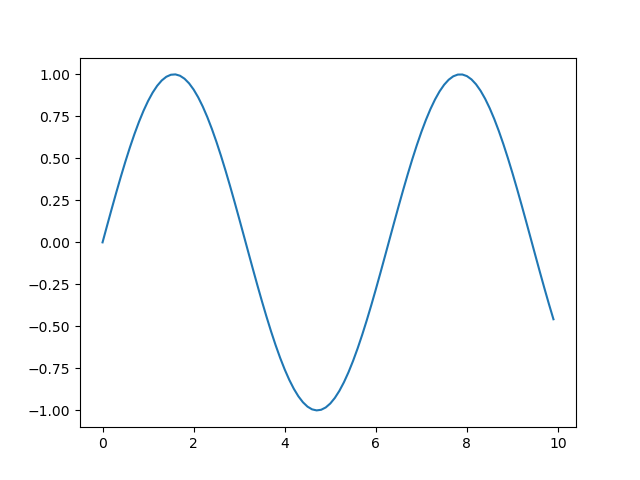# Change Figure Size in MatplotlibChange Figure Size in Matplotlib. In this tutorial, we'll take a look at how to change a figure size in Matplotlib.

#### Introduction

Matplotlib is one of the most widely used data visualization libraries in Python. Much of Matplotlib's popularity comes from its customization options - you can tweak just about any element from its hierarchy of objects.

In this tutorial, we'll take a look at how to change a figure size in Matplotlib.

#### Creating a Plot

Let's first create a simple plot in a figure:

``````import matplotlib.pyplot as plt
import numpy as np

x = np.arange(0, 10, 0.1)
y = np.sin(x)

plt.plot(x, y)
plt.show()``````

The `Figure` object, if not explicitly created, is created by default and contains all the elements we can and cannot see. Changing the size of the `Figure` will in turn change the size of the observable elements too.

Let's take a look at how we can change the figure size.

## Applied Data Science with Python Certification Training Course -IgmGuru

Master Applied Data Science with Python and get noticed by the top Hiring Companies with IgmGuru's Data Science with Python Certification Program. Enroll Now

## 50 Data Science Jobs That Opened Just Last Week

Data Science and Analytics market evolves to adapt to the constantly changing economic and business environments. Our latest survey report suggests that as the overall Data Science and Analytics market evolves to adapt to the constantly changing economic and business environments, data scientists and AI practitioners should be aware of the skills and tools that the broader community is working on. A good grip in these skills will further help data science enthusiasts to get the best jobs that various industries in their data science functions are offering.

## Basic Data Types in Python | Python Web Development For Beginners

In the programming world, Data types play an important role. Each Variable is stored in different data types and responsible for various functions. Python had two different objects, and They are mutable and immutable objects.

## Data Visualization With Python: Matplotlib

Data visualization is the graphical representation of data in a graph, chart or other visual formats. It shows relationships of the data with images.

## Data Science With Python | Python For Data Science | Data Science For Beginners

This Data Science with Python Tutorial will help you understand what is Data Science, basics of Python for data analysis, why learn Python, how to install Python, Python libraries for data analysis, exploratory analysis using Pandas, introduction to series and dataframe, loan prediction problem, data wrangling using Pandas, building a predictive model using Scikit-Learn and implementing logistic regression model using Python.